## General Question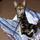# How can I factorise this...

Asked by Tennis5tar (1263) February 26th, 2008

5y²+4y-29=0

I’m having trouble with it seeing as 29 is a prime number.

At (5y___)(y____) so far, can anyone give me a hand?

Observing members: 0Composing members: 0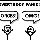first of all, correct term is to factor, not to factorise.

Secondly, while you are right that because 29 is a prime number this cannot be easily factored, you can always use the quadratic formula…

ay^2 + by + c

y = [negb +/- sqrt(b^2–4ac)]/2a

in this case, [neg4 +/- sqrt(16 + 145)]/10

=neg4/10 +/- sqrt(1.61)...

this formula gives you the factors of the equation, what y= such that the equation holds…you can use this to factor the equation.

jdvanderk (155)“Great Answer” (2) Flag as…In England, the term is to factorise. This is part of a simultanious equation question using (I think) substitution.
If it helps at all, the question appears in a C1 paper in the Edexcel AS-Level (non-calculator, which makes me think it’s not using the formula mentioned).

Tennis5tar (1263)“Great Answer” (0) Flag as…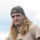If you think of it as (5y + a)(y + b) + c, there are infinite possibilities for a, b, and c such as {9, -1, 20}

Perchik (4992)“Great Answer” (0) Flag as…I only need a and b though, as I need to find two seperate values for y.

Tennis5tar (1263)“Great Answer” (0) Flag as…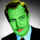I’ve tried it a few times and yes, that 29 is a dirty bugger. Using the quadratic formula jdvanderk mentioned, however, you can get a solution. Here in Canada I’ve done high school calculus courses where you’re expected to have the quadratic formula memorized so I could see it appearing on a non-calculator test.

paulc (2924)“Great Answer” (0) Flag as…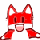(5y-1)(y+1)-28

skwerl88 (532)“Great Answer” (0) Flag as…That is what I was suggesting skwerl, but that could be any arrangement of numbers.

Perchik (4992)“Great Answer” (0) Flag as…if its part of a simultaneous equation question, do you have the other equation?

if its not, you could use the completing the square trick,

5y^2 + 4y – 29=0
y^2 + 4/5y = 29
y^2 + 4/5y + 4/25 = 29 + 4/25 where 4/25 is (b/2)^2
(y+2/5)^2 = 29 + 4/25

if you square root both sides you can solve for both answers, if you assume the square root of the right side is +/-

again though, this will not give you a nice answer (i dont think), unless 29*25 + 4 is a nice square

jdvanderk (155)“Great Answer” (0) Flag as…Actaully, 29*25 + 4 = 729 = 27^2

Perchik (4992)“Great Answer” (0) Flag as…thanks Perchik!

well then, the equation becomes

(y+2/5)^2 = 729/25

y + 2/5 = +/-27/5

y = -29/5 and 25/5

which means that the original equation factors into

(y-5)(y+29/5) = 0
or
5*(y-5)*(y+29/5) = 0

jdvanderk (155)“Great Answer” (0) Flag as…My hands are up… I’m an idiot. I lost a 1 in my calculations.

It should have been 5y^+4y-28=0

Really sorry you guys. Thank you so much anyway!

Tennis5tar (1263)“Great Answer” (0) Flag as…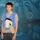How can you Factor something? Its not even a verb, it’s an improper noun! Now, to Factorise, there’s something we can all enjoy!

bluemukaki (4332)“Great Answer” (0) Flag as…Response moderated (Spam)

or